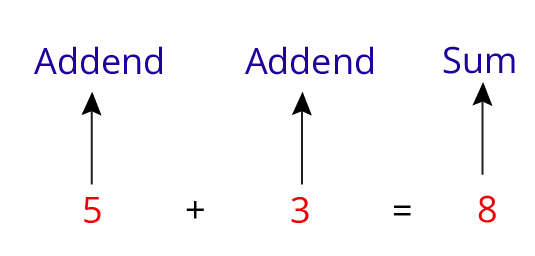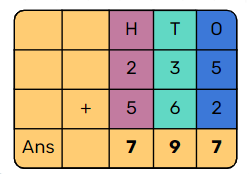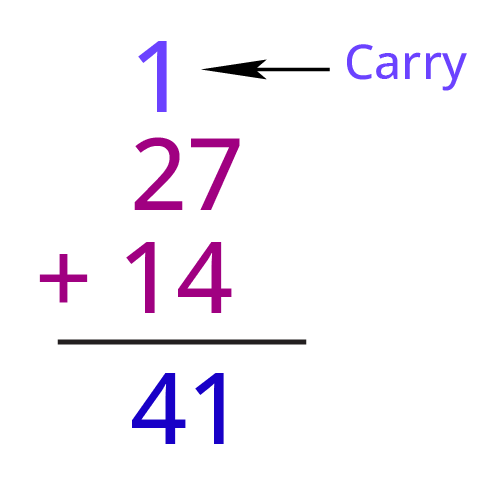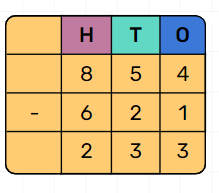Courses
Courses for Kids
Free study material
Free LIVE classes
More

# Fun with Give and Take Class 3 Notes CBSE Maths Chapter 6 (Free PDF Download)LIVE
Join Vedantu’s FREE Mastercalss

## NCERT Class 3 Maths Chapter 6 Fun with Give and Take Revision Notes

Class 3 Maths Fun with Give and Take is a fundamental chapter for the students. This chapter focuses on basic concepts of addition and subtraction in story methods. Students of Class 3 will be able to learn and understand all concepts of this chapter from the revision notes for Chapter 6 Give and Take prepared by our subject matter experts.

In these revision notes, the elaborate description of various concepts as given in the CBSE Class 3 Mathematics Chapter 6 are covered. With these notes, students can easily comprehend the mathematical principles and solve the sums given in the exercises. Hence, using the Give and Take revision notes will help you score better in the exams. Download the NCERT Revision Notes for Class 3 Maths Chapter 6 Fun with Give and Take for free from Vedantu.

Last updated date: 20th Sep 2023
Total views: 143.1k
Views today: 2.43k

## Revision Notes Class 3 Mathematics Chapter 2 - Give and Take

• The addition is a mathematical operation that is used to add numbers.

• Addends are the numbers that are added, i.e., the numbers to which the addition operation is applied.

•  The sum of the given numbers is the result of adding the addends.• The addition symbol is made up of one horizontal and one vertical line. It is also known as the plus sign.

• There can be more than two addends. Example:

### How to Add Two Numbers?

• Start by adding the digits in one’s place. (5 + 2 = 7)

• Then add the digits in the tens place. (3 + 6 = 9)

• Finally, add the digit in the hundreds place. (2 + 5 = 7)

• 235 + 562 = 797### Two-Digit Dddition with Carryover

Let’s understand this with an exampleAddition of Two Digit Numbers with Carryover

• Digits at one’s place are added first i.e. 7 + 4 = 11 which is 1 ten and 1 one.

• 1  ten is carried over to ten’s side.

•  Now digits at tens place are added along with carryover i.e., 2 + 1 +1 = 4 tens.

• Hence we get the final answer as 41.

### Addition by Breaking Large Numbers According to Place Value

• We can break large numbers according to their place value so that we can solve tough addition problems mentally.

Example 1 : 45 + 67

= 40 + 5 + 60 + 7

= (40 + 60) + (5 + 7)

= 100 + 12

= 112

Example 2 : 345 + 25

= 300 + 40 + 5 + 20 + 5

= (300) + (40 + 20) + (5 + 5)

= 300 + 60 + 10

= 370

### What is Subtraction?

• When we subtract one number from another it is known as subtraction.

• Subtraction is represented by the symbol (-). This symbol is also called the minus sign.

• It is used to calculate the difference between two numbers. Example: 9 - 5 = 4

### How to Subtract Two Numbers?

• Start by subtracting the digits in one’s place. (4 - 1 =3)

• Then subtract the digits in the tens place. (5 - 2 =3)

• Finally, subtract the digits in the hundreds place. (8 - 6 =2)

• 854 - 621  = 233Subtraction of Two Numbers

### Word Problems Based on Addition

• When objects from two or more collections are combined.

Example: Arya has 20 lemon sweets and 14 orange sweets. What is the total number of sweets Arya has?

In this, we will add 20 and 14 to find the total number of sweets.

Now , 20 + 14 = 34

Arya has 34 sweets.

• When there is an increase in the number.

Example: Ajay has 14 stamps. His friend gave him 23 stamps. How many stamps does Ajay have in all?

In this, we will add 14 and 23 to find a total number of stamps.

Now , 14 + 23 = 37

Ajay has 37 stamps.

• In addition to problems, the following keywords are used:

Sum, total, more, in all, added, all together.

Example:

25 and 40 more are 65 ( 25 + 40 = 65)
The sum of 19 and 44 is 63 (19 + 44 = 63)

### Word Problems Based on Subtraction

• Partitioning: When something is taken away, removed, or given away.

Example: Rita has 100 chocolates out of which few were given away to poor children. She was left with 70 chocolates, can you guess how many were given away to poor children?

If we subtract 70 from 100 we get the number of chocolates given away to poor children.

100 - 70 = 30.

Hence 30 chocolates were given away to poor children.

• Reducing: When we have to find how much is left or how much is removed.

Example: Ajay had Rs 1000. He spent Rs 200 on groceries. Calculate the amount of money he has now.

If we subtract 200 from 1000 we get the amount of money he is left with.

1000 - 200 = 800

Hence, Ajay is left with Rs 800.

• Comparison: When we have to compare two quantities (more than/ less than)

Example: Rahul has 80 candies and Aman has 45 candies. Which person has more candies and by how much.

Clearly, Rahul has more candies than Aman.

To find how many more candies Rahul has we will subtract 45 from 80

80 - 45 = 35

Hence Rahul has 35 more candies than Aman.

• In subtraction problems, the following keywords are used:

Take away, how many more, how many less, how many left, reducing, greater, smaller, less than

Example :

3 less than 34 is 31 ( 34 - 3  = 31)
Reducing 100 by 34 gives 66 ( 100 -34 = 66)

### Practice Questions

Question 1. Solve the following

• 67 + 9 + 8

• 80 + 150

• 345 - 20

Question 2. A teacher noted the choice of fruits of her students in the following table.

 Students Mangoes Strawberries Girls 40 15 Boys 60 10 Total

### Complete the Above Table and Answer the Following Questions:

• How many students in the school like strawberries?

• How many students in the school like mangoes?

• 84

• 230

• 325

 Students Mangoes Strawberries Girls 40 15 Boys 60 10 Total 100 25
• 25

• 100

## Importance of NCERT Class 3 Chapter 6 Maths Give and Take Revision Notes

Here is a list of reasons why Fun with Give and Take Class 3 Maths revision notes are the ideal study resource for your exam preparation.

• The sums of this chapter have been explained in a simple language. Students can read and understand the methods of solving the sums easily from these revision notes.

• Understand the use of words in the sums of this chapter. You will be able to understand what is asked in a sum instantly using these revision notes so, you can solve the sums of this chapter easily. For instance, you will be able to comprehend what reducing 98 by 34 gives and will be easily able to solve similar sums in no time.

• Learn how to solve all types of sums covered in the chapter with the help of these revision notes.

• The objective of introducing this chapter in the curriculum will be clear to the students. They will learn the basic concepts of addition and subtraction and use them to solve arithmetic problems efficiently.

Students will imbibe the concept of addition and subtraction in arithmetic. The explanation of the sums will help them realize the applications of these concepts. They will be able to understand the meaning of ‘23 less than 89 is’ and solve all sums covered in the chapter with the help of these revision notes easily.

## Key Features of Revision Notes for Class 3 Maths Chapter 6 Fun with Give and Take

CBSE Class 3 students will be benefitted by using the revision notes for Maths Chapter 6 Give and Take in the following ways.

• Top-quality revision notes from subject experts with the highest precision

• In accordance with CBSE Class 3 Mathematics curriculum

• Easy explanations for the conceptual development of the young minds

• Ensuring a complete understanding of the basic principles of addition and subtraction

• Provides an easy way to revise the fundamental concepts of the chapter before the exam

• Covers basic tips from the experts to solve the exercise problems for better scores in the exams

## Conclusion

Download the revision notes for NCERT Class 3 Chapter 6 Maths Fun with Given and Take in PDF format from Vedantu for free. Refer to these revision notes to understand the meaning of the 26 62 puzzle and other similar arithmetic problems to score better in your exams.

## FAQs on Fun with Give and Take Class 3 Notes CBSE Maths Chapter 6 (Free PDF Download)

1. Are the revision notes for Class 3 Maths Chapter 6 PDF free?

You can download the revision notes PDF for free from the official website and mobile app of Vedantu and use it as a reference for your exam preparation.

2. How can I prepare the concepts for Chapter 6 Maths before an exam?

By learning the simpler description of the fundamental principles in these revision notes, you can thoroughly prepare this chapter before your Maths exam.

3. What are the tips to understand the sums in Chapter 6 Maths Class 3?

Follow the Solutions and revision notes of the arithmetic problems given on Vedantu for a good understanding of this chapter. You will find a pattern to understand how to solve these questions with the help of the study materials provided on Vedantu.